# Printable Grids SquaresOtherApr 01, 2021By PrintableeNo Comment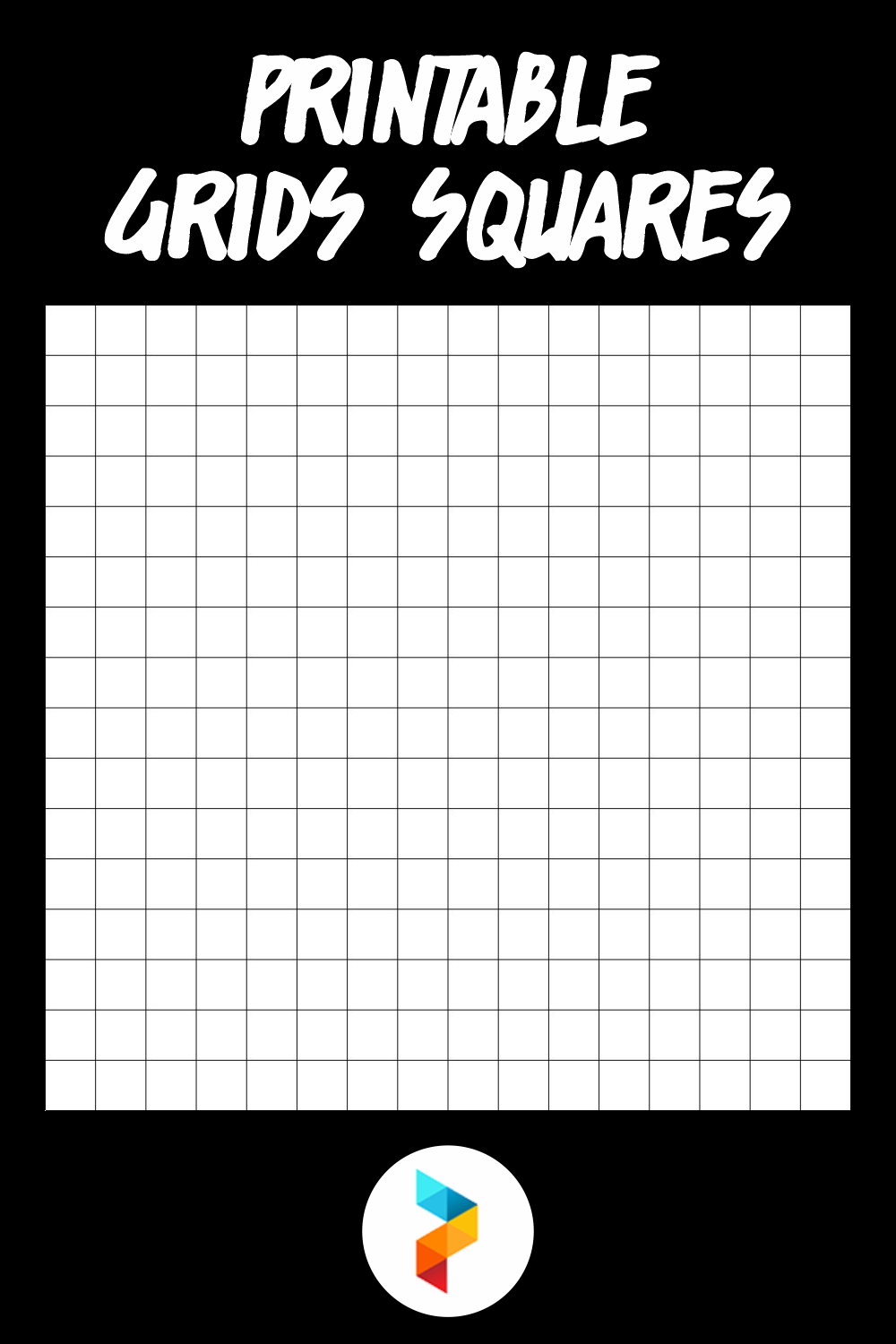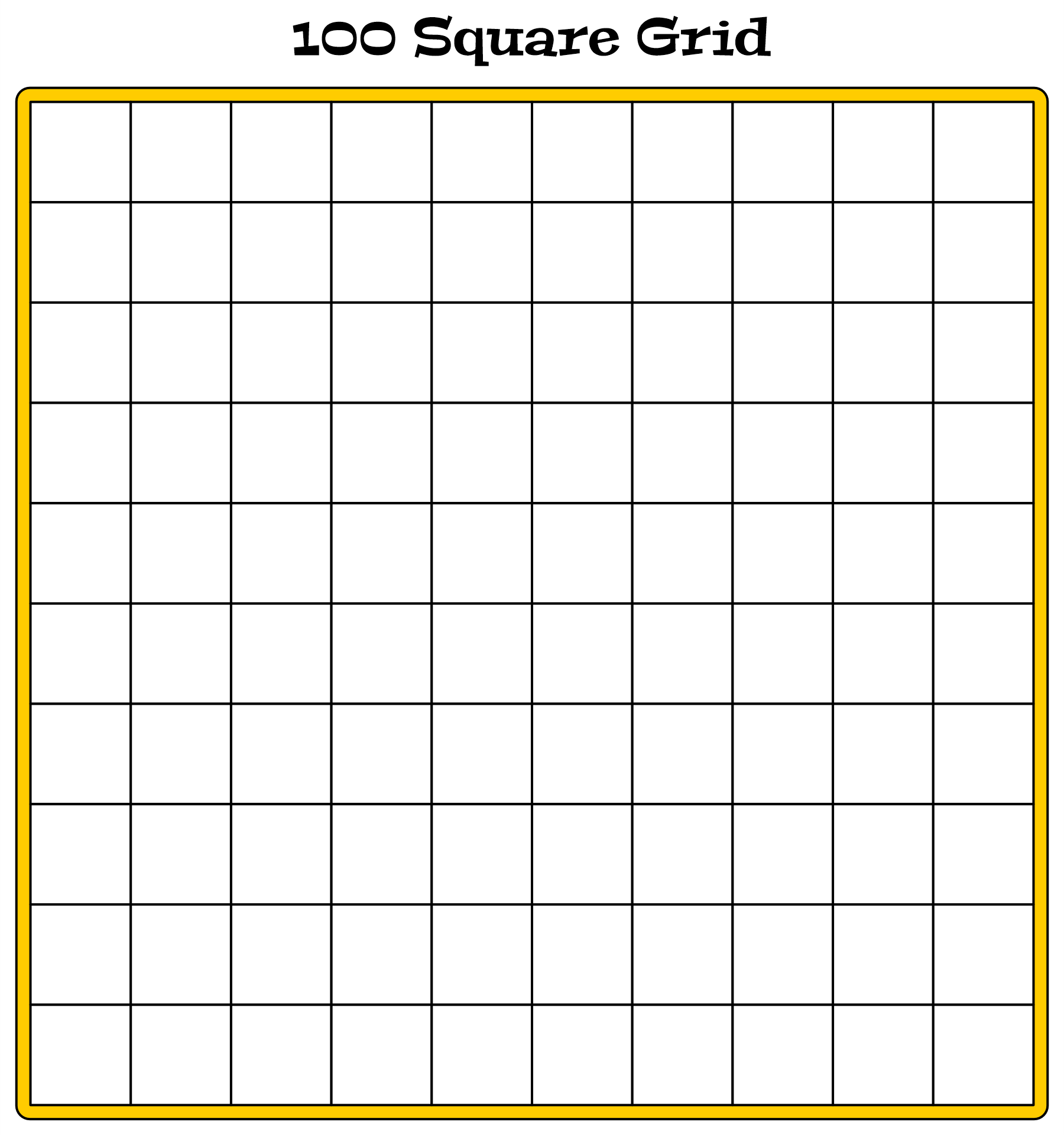### What are grid squares?

They are simple squares that are arranged sequentially in rows and columns. Every corner that meets in each box forms dots called intersections. The format is usually written as numbers of rows x numbers of columns, like 5 x 6.

### What are the functions of grid squares?

1.       Draws 2D and 3D shapes in maths easier

To get precise measurements, grid squares help us to count how many boxes we need to make outlines for.

2.       An aid for working on coordinate points

In mathematics, we may find a Cartesian coordinate system. We are required to create X and Y axes which are horizontal and vertical lines. When we need to put a certain coordinate point, it’ll be easier to use grid squares because it has dots from intersections between each corner of boxes.

3.       Helps drawing with tracing method

When we want to draw using the tracing method, instead of overlaying the blank sheets on the pictures, we can use the grid squares worksheet. So, we can follow the shapes of the lines inside the squares.

4.       A medium for number charts

Grid squares simplify the separation of each number in charts. This grid also helps to find certain patterns of operations. For instance, we may find diagonal lines when finding multiplication results.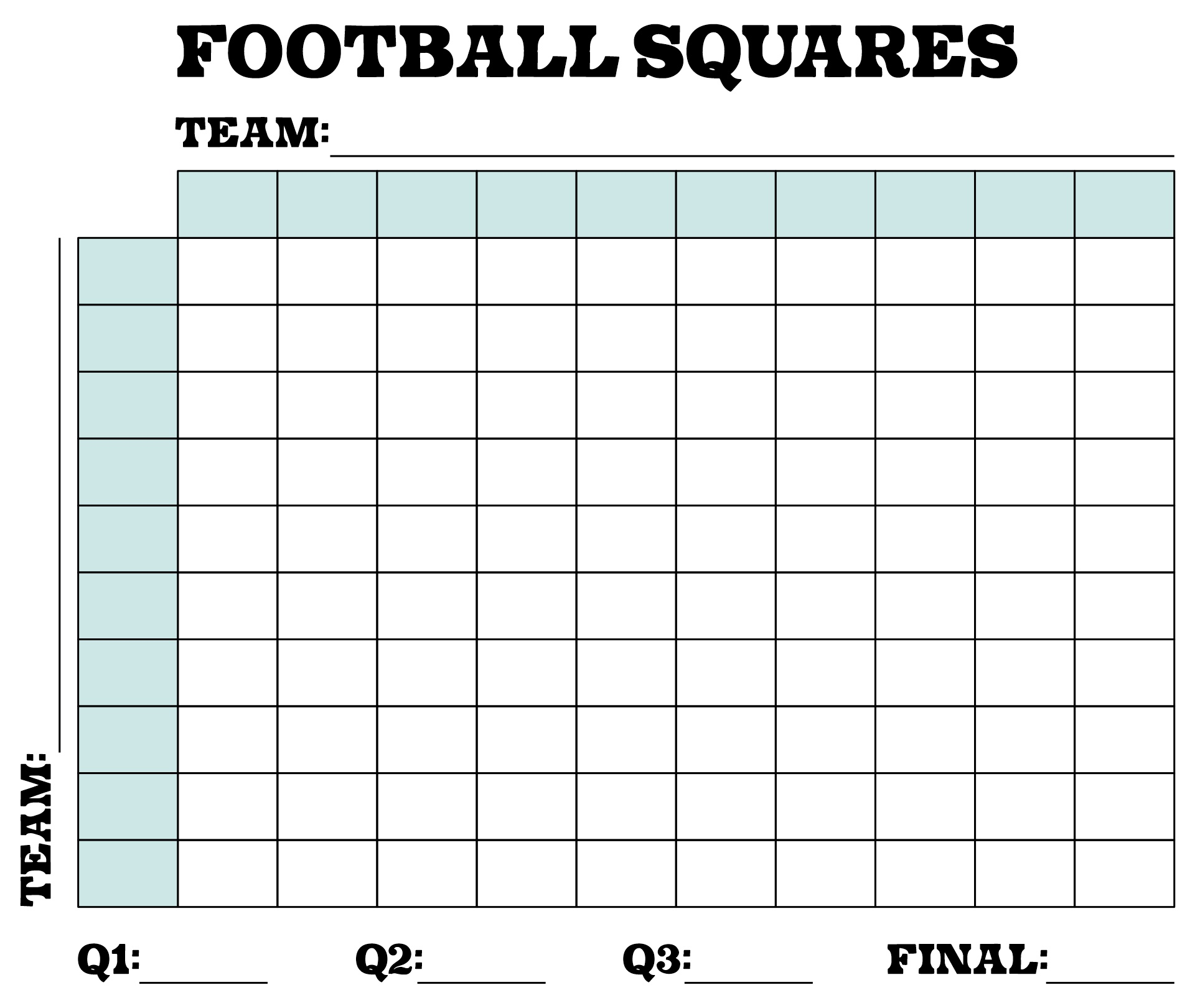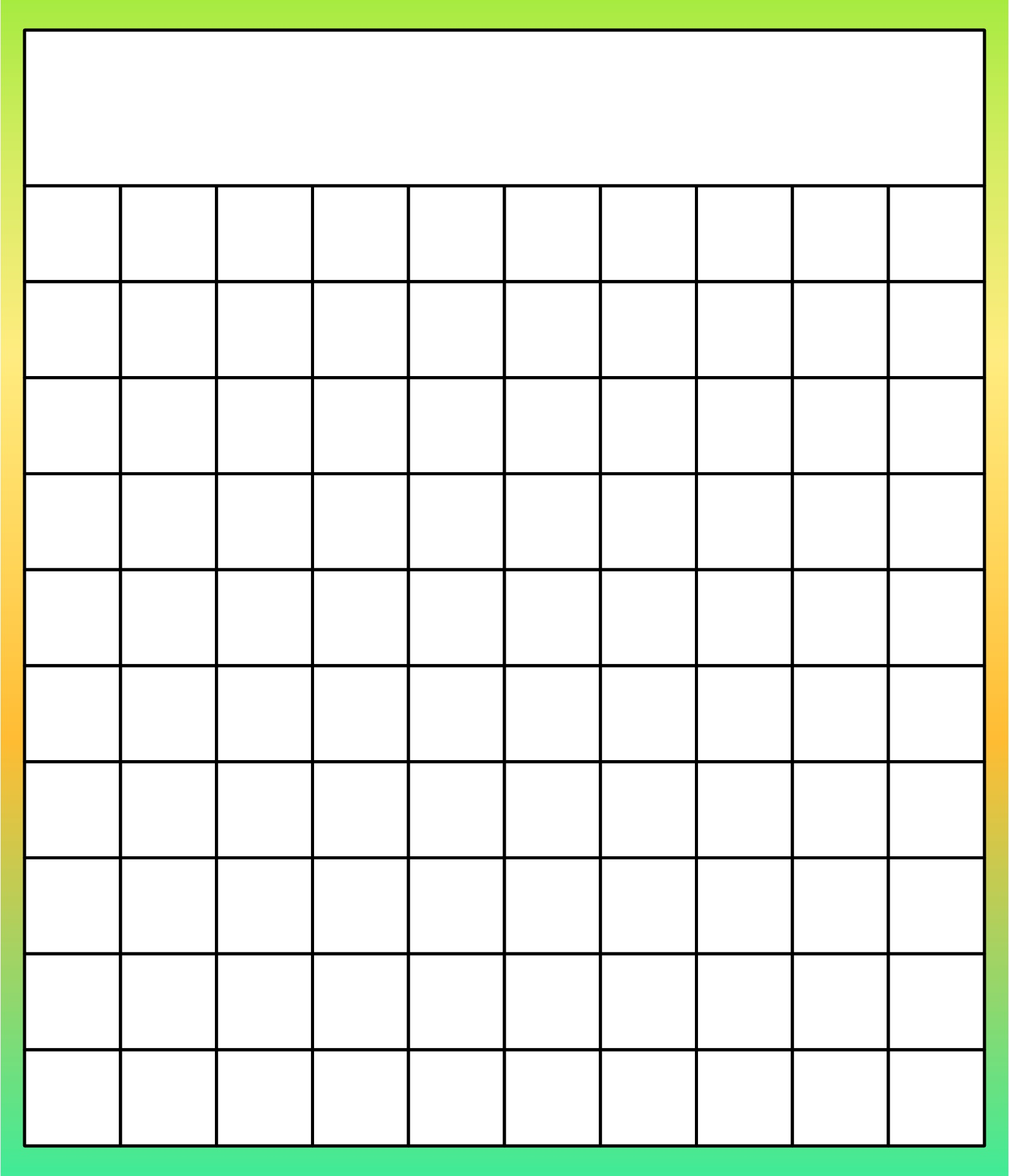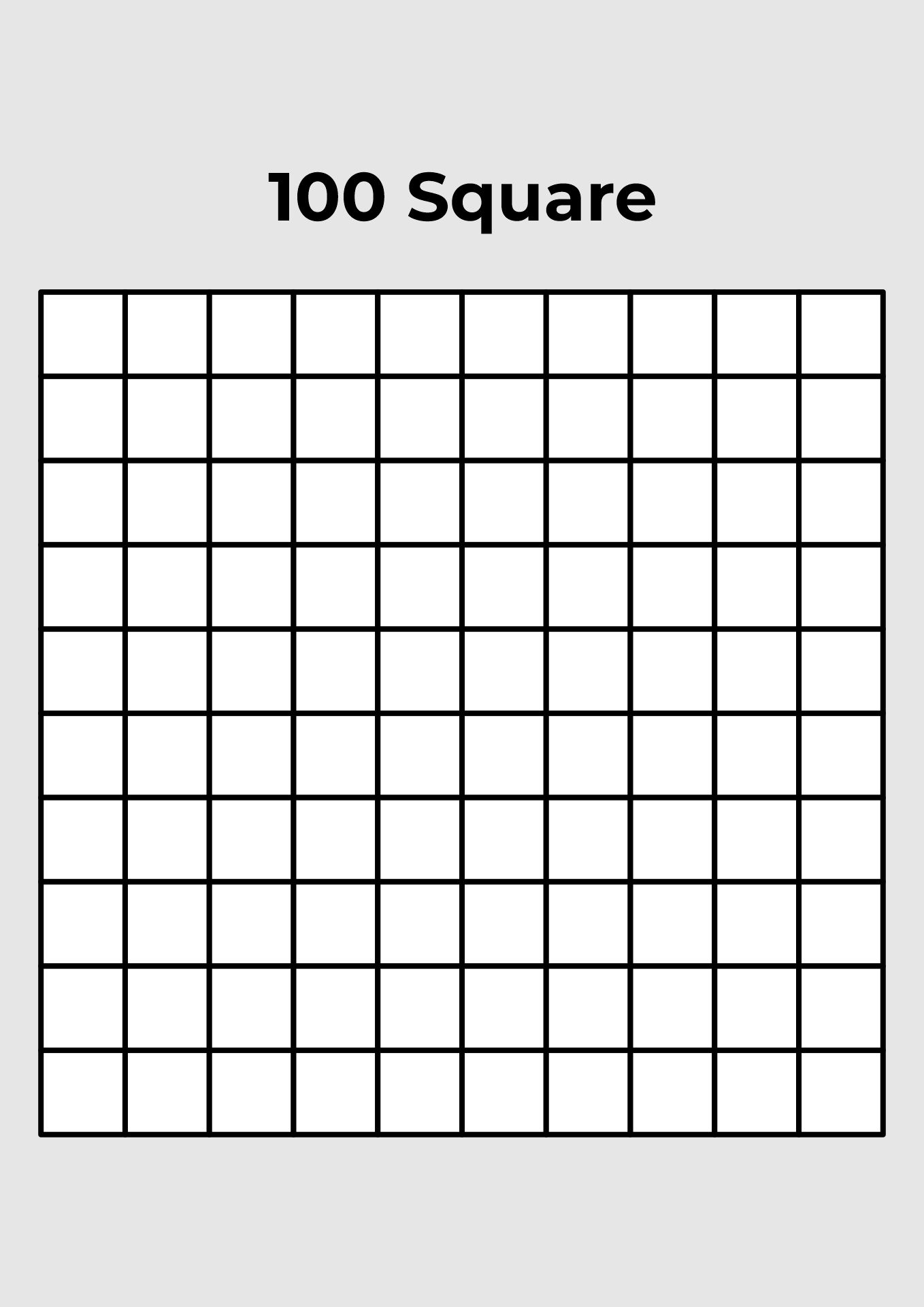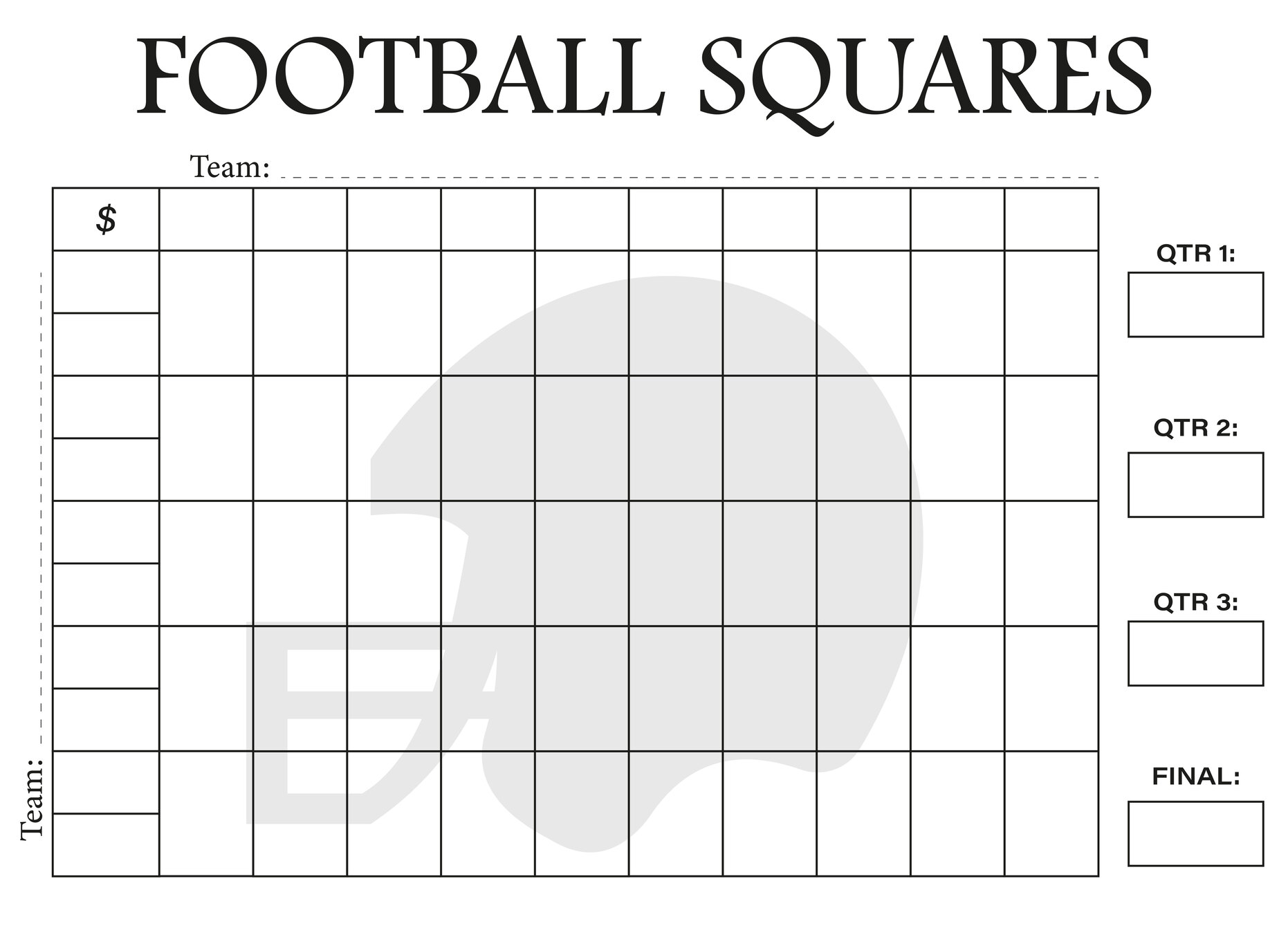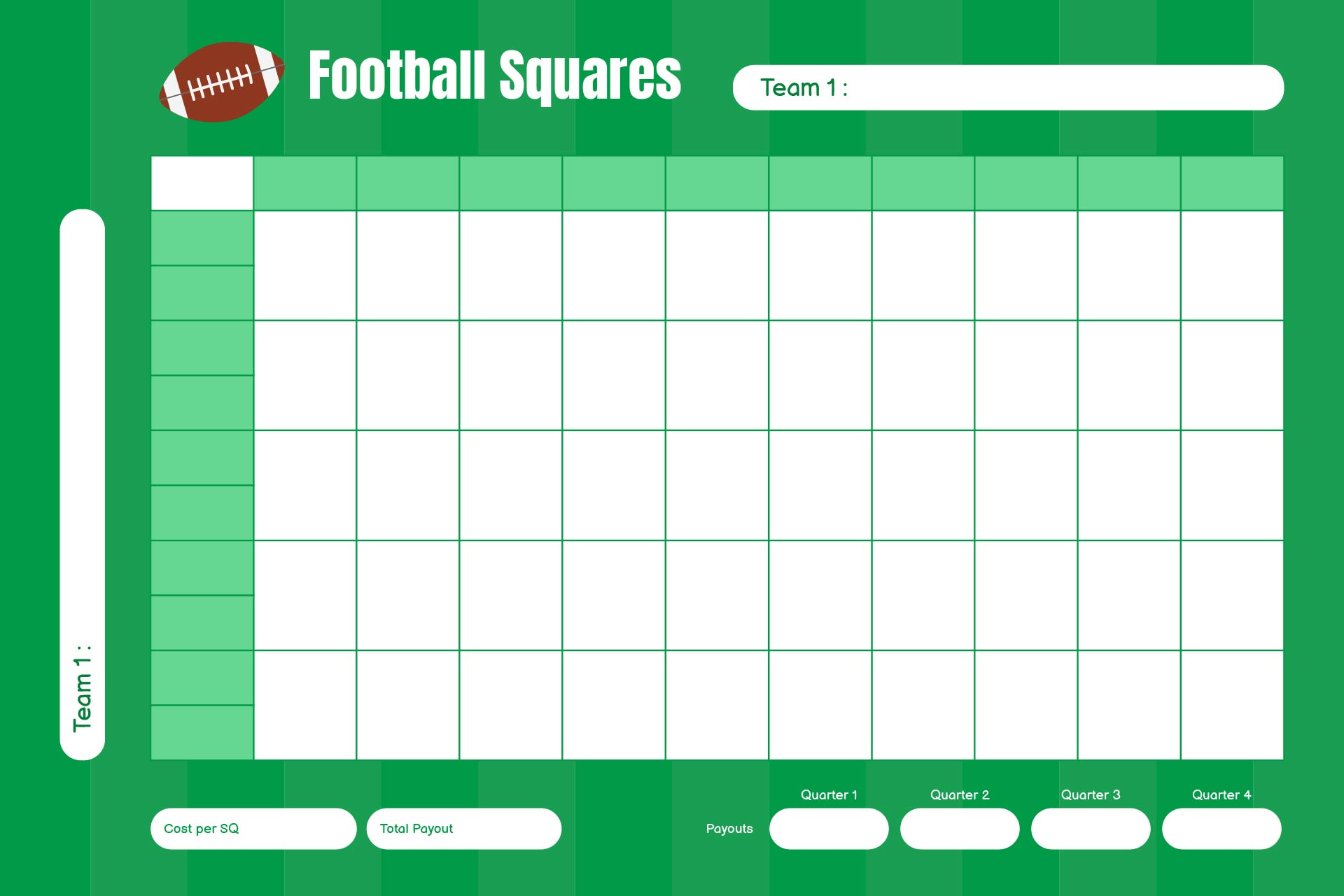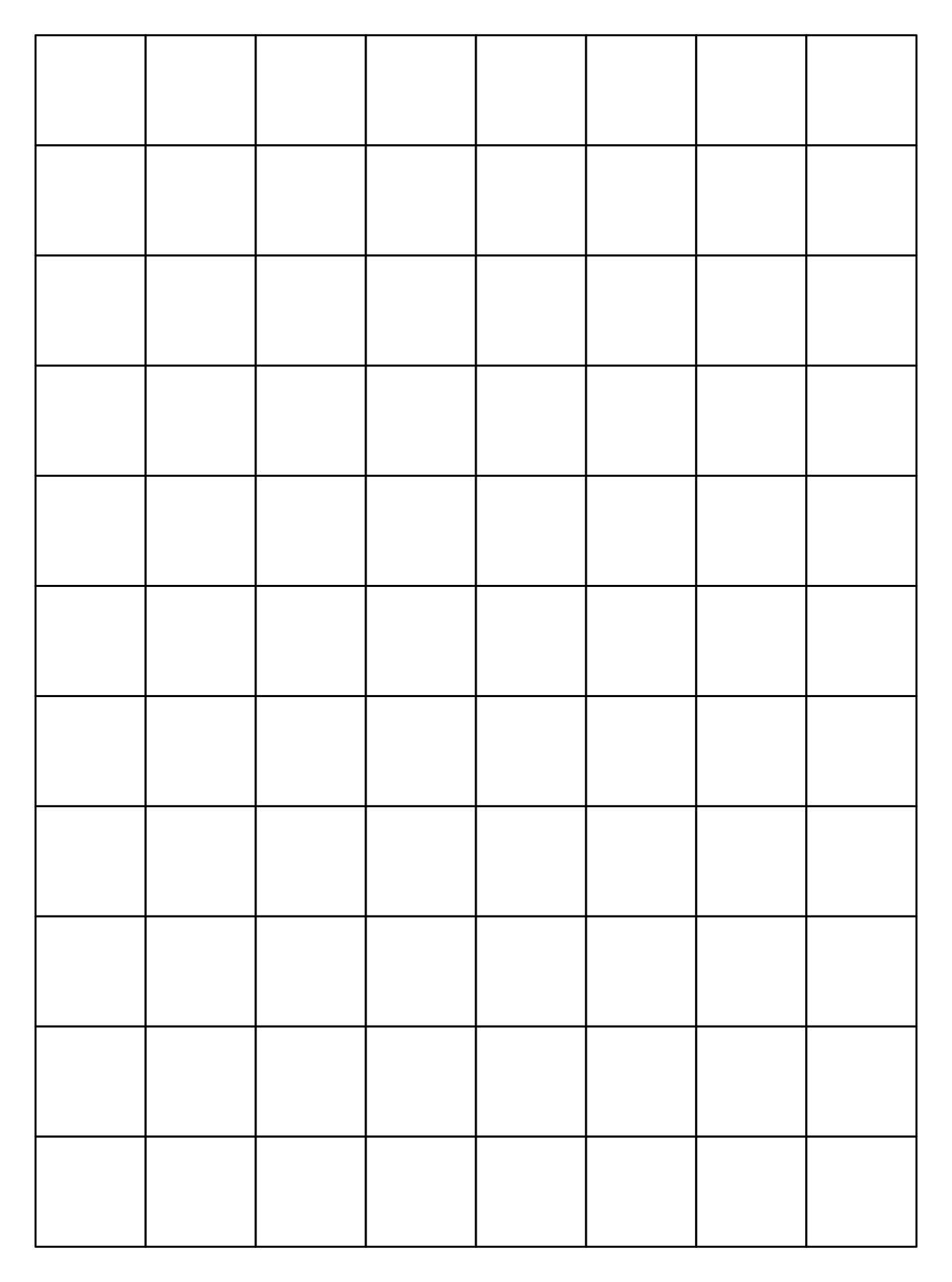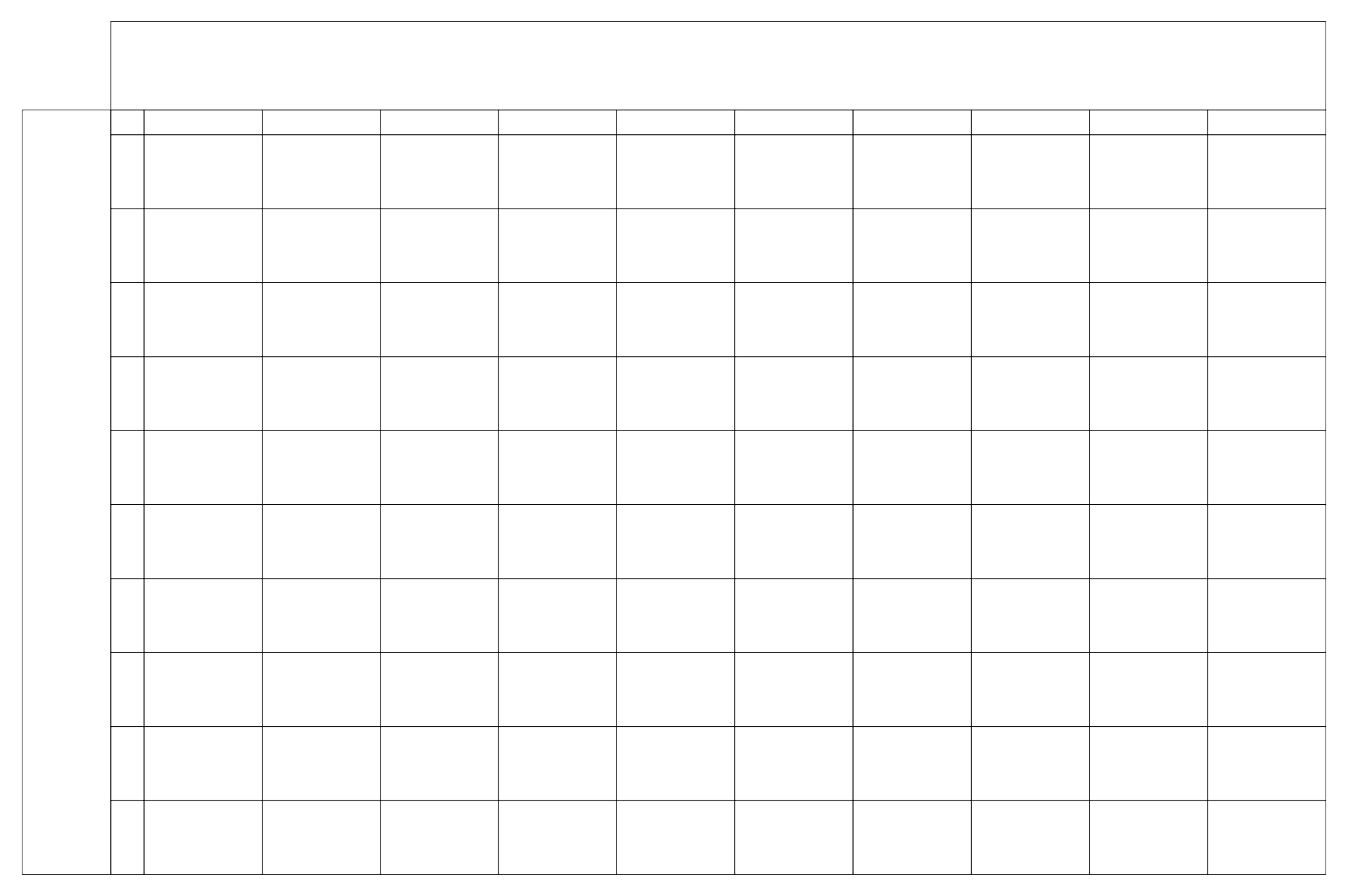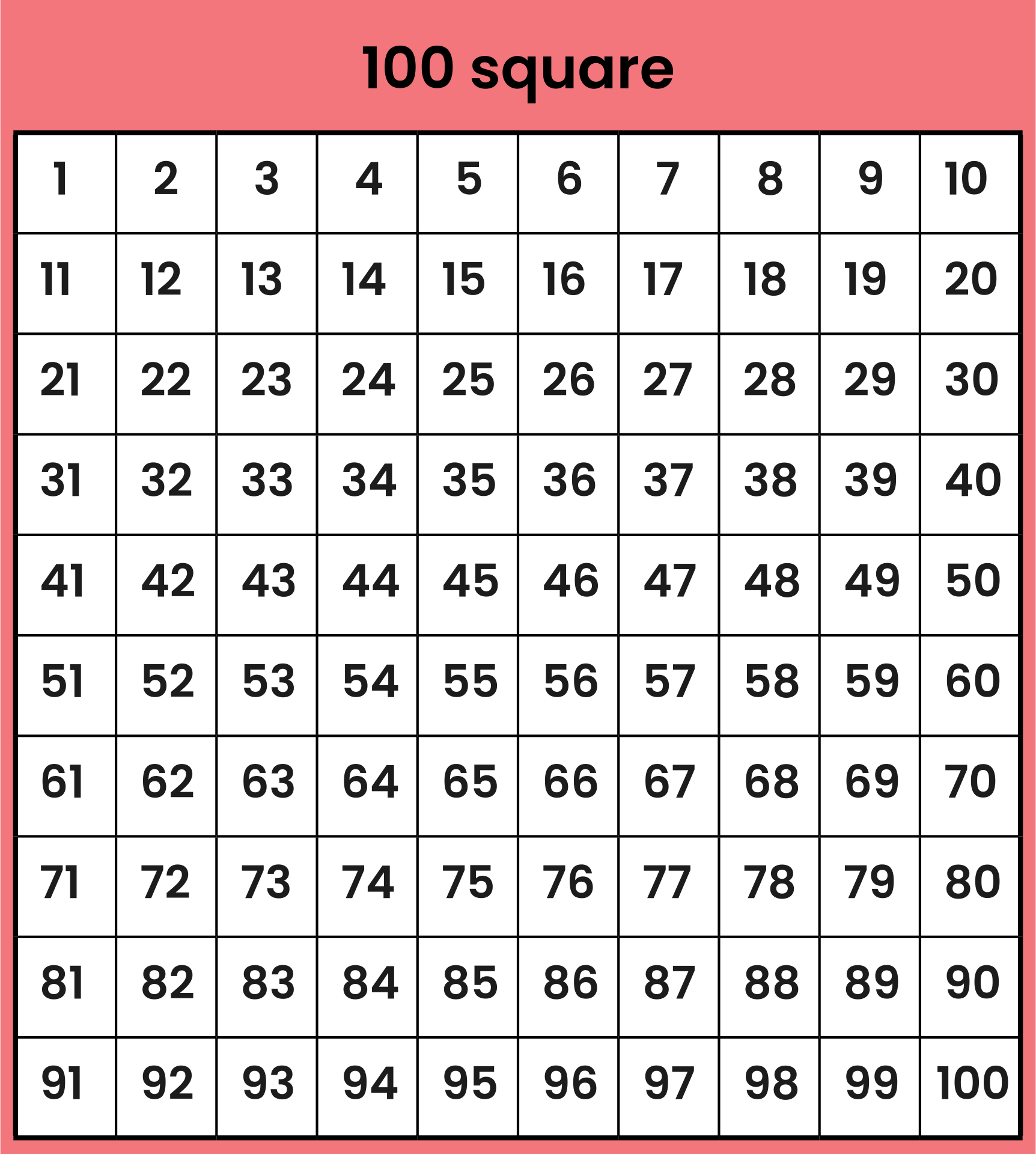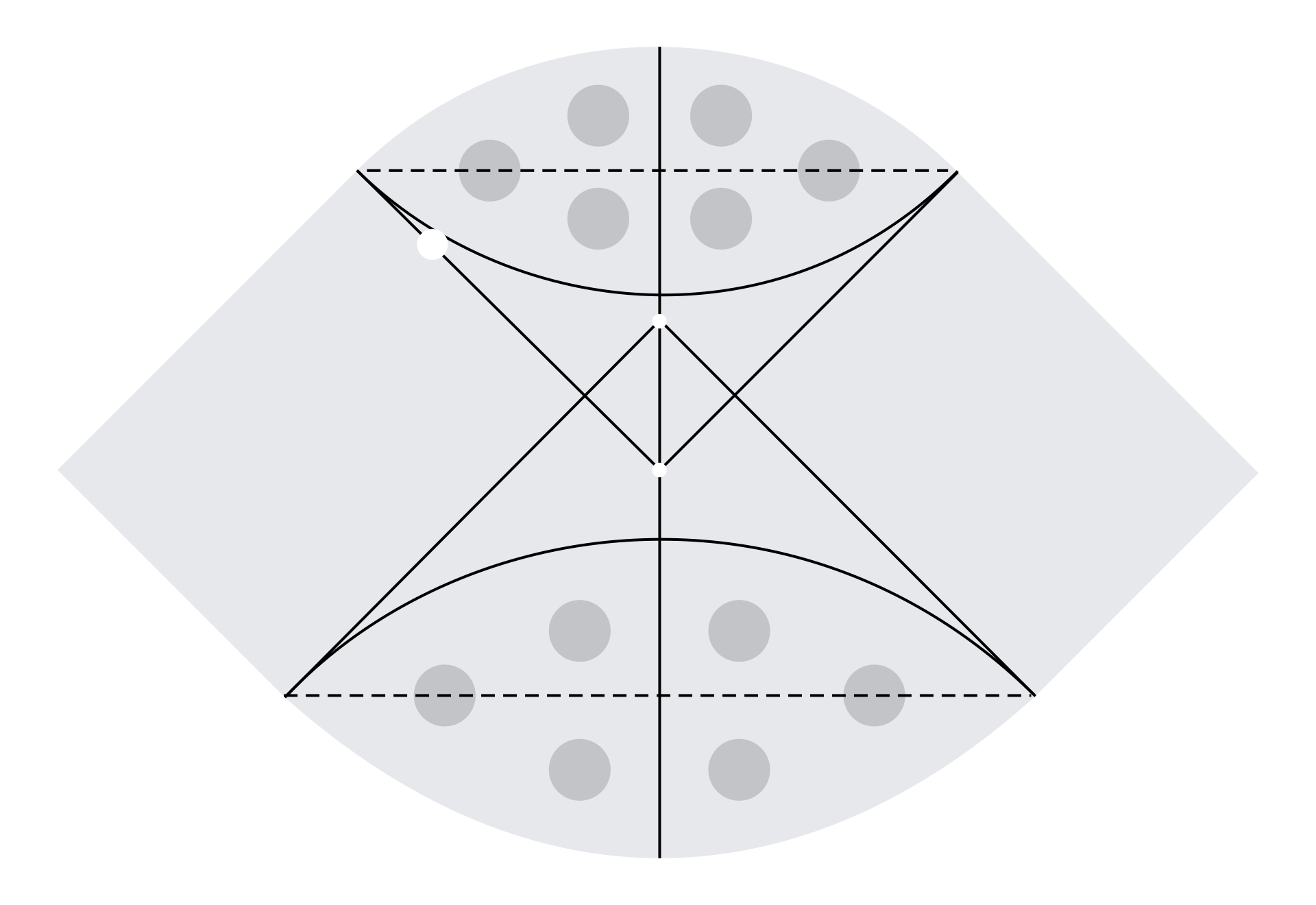### How to use grid squares?

1.       For drawing 2D or 3D shapes

If we want to draw a square, make sure the boxes we’ll need for guidance have the same amount. If we use four squares to create a line, then we need to do the same for the other sides. This requirement is also applied when drawing a cube. Double check that each length, width and height use the same number of boxes.

2.       For solving Cartesian coordinate

Create X and Y axes horizontally and vertically. Make sure both lines have intersections. Every point on the top right quadrant has positive values and vice versa. When we need to solve (4, 6) coordinates, for instance, we can look up the top right side since those have positive values. Then, point coordinate 4 on X axis and 6 on Y axis.

3.       For tracing method

Count how many squares to start. Instead of seeing a whole outline of a drawing, we can see the shapes of the lines inside the box. Draw one by one until we see a drawing.

4.       For a hundreds chart

Simply draw 10 x 10 grid squares and put the numbers from 1 to 100. Play with it to find patterns of operations.